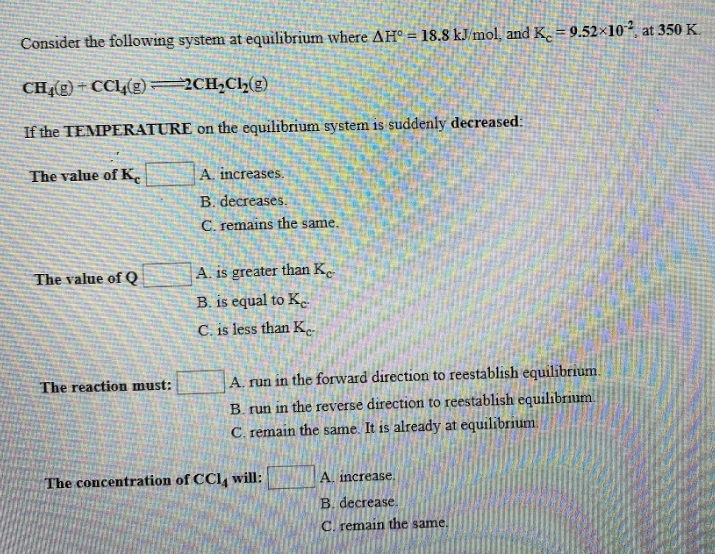# Consider the following system at equilibrium where ΔH° = -18.8 kJ/mol, and Kc = 9.52x10^-2, at 350 K. CH4(g) + CCl4(g) ⇌ 2Ch2Cl2(g) If the TEMPERATURE on the equilibrium system is suddenly decreased. The value of Kc____ a) increases b) decreases c) remains the same The value of Q ____ a) is greater than Kc b) is equal to Kc c) is less than Kc The reaction must: The concentration of CCl4 will: a) increase b) decrease c) remain the same